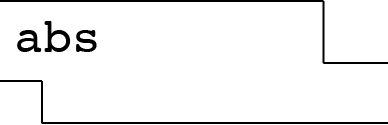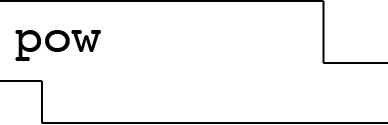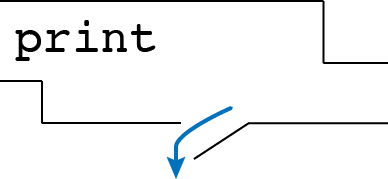# Control

Tips for navigating the slides:
• Press O or Escape for overview mode.
• Visit this link for a nice printable version
• Press the copy icon on the upper right of code blocks to copy the code

### Class outline:

• Side effects
• More function features
• Conditionals
• Booleans
• Iteration

## Side effects

### The None value

The special value `None` represents nothingness in Python.

Any function that doesn't explicitly return a value will return `None`:

``````
def square_it(x):
x * x
``````

When a function returns `None`, the console shows no output at all:

``````
square_it(4)
``````

Attempting to treat the `None` like a number will result in an error:

``````
sixteen = square_it(4)
sum = sixteen + 4     # 🚫 TypeError!
``````

### Side effects

A side effect is when something happens as a result of calling a function besides just returning a value.

The most common side effect is logging to the console, via the built-in `print()` function.

``````
print(-2)
``````

A similar side effect is writing to a file:

``````
f = open('songs.txt', 'w')
f.write("Dancing On My Own, Robyn")
f.close()
``````

### Side effects vs. Return values

``````
def square_num1(number):
return pow(number, 2)
``````
``````
def square_num2(number):
print(number ** 2)
``````
• Which one has a side effect?
The second function has a side effect, because it prints to the console.

• What data type do they each return?
The first function returns a number, the second one returns `None`.

### Pure vs. non-pure functions

Arguments Return value
Pure functions
just return values.
-22
2, 101024
Non-pure functions
have side effects.
-2Python displays output "-2"
`None`

### Nested print statements

What will this display?

``````
print(print(1), print(2))
``````

## More function features

### Default arguments

In the function signature, a parameter can specify a default value. If that argument isn't passed in, the default value is used instead.

``````
def calculate_dog_age(human_years, multiplier = 7):
return human_years * multiplier
``````

These two lines of code have the same result:

``````
calculate_dog_age(3)
calculate_dog_age(3, 7)
``````

Default arguments can be overriden two ways:

``````
calculate_dog_age(3, 6)
calculate_dog_age(3, multiplier=6)
``````

### Multiple return values

A function can specify multiple return values, separated by commas.

``````
def divide_exact(n, d):
quotient = n // d
remainder = n % d
return quotient, remainder
``````

Any code that calls that function must also "unpack it" using commas:

``````
q, r = divide_exact(618, 10)
``````

### Doctests

Doctests check the input/output of functions.

``````
def divide_exact(n, d):
"""
>>> q, r = divide_exact(2021, 10)
>>> q
202
>>> r
1
"""
quotient = n // d
remainder = n % d
return quotient, remainder
``````

See more in Python doctests documentation.

## Boolean expressions

### Booleans

A Boolean value is either `True` or `False` and is used frequently in computer programs.

Google Maps uses a boolean to decide whether to avoid highways in driving directions:

``````
avoid_highways = True
``````

Twitter uses a boolean to remember where the user allows personalized ads:

``````
``````

### Boolean expressions

An expression can evaluate to a Boolean. Most Boolean expressions use either comparison or logical operators.

An expression with a comparison operator:

``````
``````

An expression with a logical operator:

``````
wear_jacket = is_raining or is_windy
``````

### Comparison operators

 Operator Meaning True expressions == Equality `32 == 32` != Inequality `30 != 32` > Greater than `60 >= 32 ` >= Greater than or equal `60 >= 32 `, `32 >= 32` < Less than `20 < 32` <= Less than or equal `20 < 32`, `32 <= 32`

⚠️ Common mistake: Do not confuse `=` (the assignment operator) with `==` (the equality operator).

### Logical operators

OperatorTrue expressions Meaning
`and` `4 > 0 and -2 < 0` Evaluates to `True` if both conditions are true. If one is `False` evaluates to `False`.
`or` `4 > 0 or -2 > 0 ` Evaluates to `True` if either condition is true. Evaluates to `False` only if both are false.
`not` `not (5 == 0)` Evaluates to `True` if condition is false; evaluates to `False` if condition is true.

### Compound booleans

When combining multiple operators in a single expression, use parentheses to group:

``````
may_have_mobility_issues = (age >= 0 and age < 2)  or age > 90
``````

### Boolean expressions in functions

A function can use a Boolean expression to return a result based on the values of the parameters.

``````
``````
``````
def should_wear_jacket(is_rainy, is_windy):
return is_rainy or is_windy
``````

### Exercise

These are un-graded exercises you can do after the lecture to make sure you grok the basics:

## Statements

### Statements

A statement is executed by the interpreter to perform an action.

So far we've seen a few...

Statement type Example
Assignment statement
``````
name = 'sosuke'
greeting = 'ahoy, ' + name
``````
Def statement
``````
def greet(name):
return 'ahoy, ' + name
``````
Return statement
``````
return 'ahoy, ' + name
``````

### Compound statements

A compound statement contains groups of other statements.

``````
<statement>   # SUITE
<statement>
...
<statement>   # SUITE
<statement>
...
``````

The first header determines a statement's type, and the header of each clause controls the suite that follows.

### Execution of suites

A suite is a sequence of statements.

``````
<statement>   # SUITE
<statement>
...
<statement>   # SUITE
<statement>
...
``````

Execution rule for a sequence of statements:

• Execute the first statement
• Unless directed otherwise, execute the rest

## Conditional statements

### Conditional statements

A conditional statement gives your code a way to execute a different suite of code statements based on whether certain conditions are true or false.

``````
if <condition>:
<statement>
<statement>
...
``````

A simple conditional:

``````
clothing = "shirt"

if temperature < 32:
clothing = "jacket"
``````

### Compound conditionals

A conditional can include any number of `elif` statements to check other conditions.

``````
if <condition>:
<statement>
...
elif <condition>:
<statement>
...
elif <condition>:
<statement>
...
``````
``````
clothing = "shirt"

if temperature < 0:
clothing = "snowsuit"
elif temperature < 32:
clothing = "jacket"
``````

### The else statement

A conditional can include an `else` to specify code to execute if no previous conditions are true.

``````
if <condition>:
<statement>
...
elif <condition>:
<statement>
...
else <condition>:
<statement>
...
``````
``````
if temperature < 0:
clothing = "snowsuit"
elif temperature < 32:
clothing = "jacket"
else:
clothing = "shirt"
``````

### Conditional statements summary

``````
if num < 0:
sign = "negative"
elif num > 0:
sign = "positive"
else:
sign = "neutral"
``````

Syntax tips:

• Always start with `if` clause.
• Zero or more `elif` clauses.
• Zero or one `else` clause, always at the end.

### Execution of conditional statements

Each clause is considered in order.

• If it's true, execute the suite of statements underneath and skip the remaining clauses.
• Otherwise, continue to the next clause.### Conditionals in functions

It's common for a conditional to be based on the value of the parameters to a function.

``````
def get_number_sign(num):
if num < 0:
sign = "negative"
elif num > 0:
sign = "positive"
else:
sign = "neutral"
return sign
``````
``````
get_number_sign(50)  # "positive"
get_number_sign(-1)  # "negative"
get_number_sign(0)   # "neutral"
``````

### Returns inside conditionals

A branch of a conditional can end in a return, which exits the function entirely.

``````
def get_number_sign(num):
if num < 0:
return "negative"
elif num > 0:
return "positive"
else:
return "neutral"
``````
``````
get_number_sign(50)  # "positive"
get_number_sign(-1)  # "negative"
get_number_sign(0)   # "neutral"
``````

### Exercise

These are un-graded exercises you can do after the lecture to make sure you grok the basics:

## While loops

### Why loops?

Consider the following code...

``````
print(9 * 1)
print(9 * 2)
print(9 * 3)
print(9 * 4)
print(9 * 5)
``````

This code is repetitive and variable, but the variability is sequential - it just adds one to the right-hand side number each time. In English, we could describe this as "print out the first 5 multiples of 9".

We can simplify this code with a loop!

### While loops

The while loop syntax:

``````
while <condition>:
<statement>
<statement>
``````

As long as the condition is true, the statements below it are executed.

``````
multiplier = 1
while multiplier <= 5:
print(9 * multiplier)
multiplier += 1
``````

The code is significantly shorter, and it can easily be extended to loop for more or less iterations.

### Changing a while loop #1

You can change the initial values of the variables used in the condition:

``````
multiplier = 3
while multiplier <= 5:
print(9 * multiplier)
multiplier += 1
``````
• What will be the first thing displayed? 27
• What will be the last thing displayed? 45
• How many iterations? 3 (for values of 3, 4, 5)

### Changing a while loop #2

You can change the condition:

``````
multiplier = 3
while multiplier <= 10:
print(9 * multiplier)
multiplier += 1
``````
• What will be the first thing displayed? 27
• What will be the last thing displayed? 90
• How many iterations? 8 (for values of 3, 4, 5, 6, 7, 8, 9, 10)

### Changing a while loop #3

You can change how much the values change between iterations:

``````
multiplier = 3
while multiplier <= 10:
print(9 * multiplier)
multiplier += 2
``````
• What will be the first thing displayed? 27
• What will be the last thing displayed? 81
• How many iterations? 4 (for values of 3, 5, 7, 9)

### Changing a while loop #4

You can change the computation inside the while loop:

``````
multiplier = 3
while multiplier <= 10:
print(10 * multiplier)
multiplier += 2
``````
• What will be the first thing displayed? 30
• What will be the last thing displayed? 90
• How many iterations? 4 (for values of 3, 5, 7, 9)

### Using a counter variable

It's common to use a counter variable whose job is keeping track of the number of iterations.

``````
total = 0
counter = 0
while counter < 5:
total += pow(2, 1)
counter += 1
``````

The counter variable may also be involved in the loop computation:

``````
total = 0
counter = 0
while counter < 5:
total += pow(2, counter)
counter += 1
``````

### Beware infinite loops

Uh oh..

``````
counter = 1
while counter < 5:
total += pow(2, counter)
``````

What one line of code would fix this?
`counter += 1`

``````
counter = 6
while counter > 5:
total += pow(2, counter)
counter += 1
``````

How do we save this code?
Intentions are unclear! Change the initial value and condition?

### Execution of loops

1. Evaluate the header’s Boolean expression.
2. If it is a true value, execute the suite of statements, then return to step 1.### Loops in functions

A loop in a function will commonly use a parameter to determine some aspect of its repetition.

``````
def sum_up_squares(start, end):
counter = start
total = 0
while counter <= end:
total += pow(counter, 2)
counter += 1

sum_up_squares(1, 5)
``````

### The break statement

To prematurely exit a loop, use the `break` statement:

``````
counter = 100
while counter < 200:
if counter % 7 == 0:
first_multiple = counter
break
counter += 1
``````

### Looping while true

If you are brave, you can write while loops like this:

``````
counter = 100
while True:
if counter % 62 == 0:
first_multiple = counter
break
counter += 1
``````

⚠️ Be very sure that you're not coding an infinite loop!

### Exercise

These are un-graded exercises you can do after the lecture to make sure you grok the basics:

### Example: Prime factors

A prime number is an integer greater than 1 whose only factors are 1 and the number itself (e.g., 3, 5, 7, 11).

``````
def is prime(n):
"""Return True iff N is prime."""
return n > 1 and smallest factor(n) == n

def smallest factor(n):
"""Returns the smallest value k>1 that evenly divides N."""
???

def print factors(n):
"""Print the prime factors of N."""
???
``````

Let's implement them together.